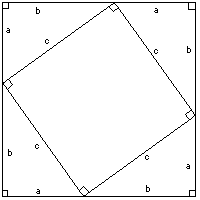# Pythagoras's theorem

a visual demonstration that for a right angled triangle,
the square of the hypotenuse is equal to the sum of the squares of the other two sides, by
Henry Bottomley.
The four blue triangles remain in the picture, so the red and yellow areas are constant, so the area of the biggest red square (on the hypotenuse) is equal to the sum of the other two red squares (on the other two sides).A proof of the theorem based on the second diagram:
the area of the big square is
(a+b)^2 , but it is also c^2 + 4.1/2.a.b,
so
a^2 + 2.a.b + b^2 = c^2 + 2.a.b , so a^2 + b^2 = c^2 .